# Geoscience Machine Learning bits and bobs – data completeness

#### 2016 Machine learning contest – Society of Exploration Geophysicists

In a previous post I showed how to use `pandas.isnull` to find out, for each well individually, if a column has any null values, and `sum` to get how many, for each column. Here is one of the examples (with more modern, pandaish syntax compared to the example in the previous post:

```for well, data in training_data.groupby('Well Name'):
print(well)
print (data.isnull().values.any())
print (data.isnull().sum(), '\n')
```

Simple and quick, the output showed met that  – for example – the well ALEXANDER D is missing 466 samples from the PE log:

```ALEXANDER D
True
Facies         0
Formation      0
Well Name      0
Depth          0
GR             0
ILD_log10      0
DeltaPHI       0
PHIND          0
PE           466
NM_M           0
RELPOS         0
dtype: int64```

A more appealing and versatile alternative, which I discovered after the contest, comes with the `matrix` function form the missingno library. With the code below I can turn each well into a Pandas DataFrame on the fly, then a missingno matrix plot.

```for well, data in training_data.groupby('Well Name'):

msno.matrix(data, color=(0., 0., 0.45))
fig = plt.gcf()
fig.set_size_inches(20, np.round(len(data)/100)) # heigth of the plot for each well reflects well length
axes=fig.get_axes()
axes.set_title(well, color=(0., 0.8, 0.), fontsize=14, ha='center')```

I find that looking at these two plots provides a very compelling and informative way to inspect data completeness, and I am wondering if they couldn’t be used to guide the strategy to deal with missing data, together with domain knowledge from petrophysics.

Interpreting the dendrogram in a top-down fashion, as suggested in the library documentation, my first thoughts are that this may suggest trying to predict missing values in a sequential fashion rather than for all logs at once. For example, looking at the largest cluster on the left, and starting from top right, I am thinking of testing use of GR to first predict missing values in RDEP, then both to predict missing values in RMED, then DTC. Then add CALI and use all logs completed so far to predict RHOB, and so on.

Naturally, this strategy will need to be tested against alternative strategies using lithology prediction accuracy. I would do that in the context of learning curves: I am imagining comparing the training and crossvalidation error first using only non NaN rows, then replace all NANs with mean, then compare separately this sequential log completing strategy with an all-in one strategy.

## From zero to JupyterLab pro on Windows 10

### Featured

N.B. last tested successfully onSepotember 27th, 2022.

I love JupyterLab, I really do! In my experience to date it proved to be the best environment for prototyping scientific computing applications interactively using Jupyter notebooks.

If you wonder if this is the right tool for you, please browse the rich documentation on the JupyterLab Interface and on how to work with Notebooks, then make sure to watch the 2018 Scipy tutorial. I guarantee that if you’ve been working with Jupyter notebooks and liked them, you will easily switch to JupyterLab and will never look back, it is only natural (also check Terraforming Jupyter to get a flavor of how much you can customize this environment to suit your needs).

Three times in the last couple of months I’ve had to make an installation from scratch on Windows 10 operated computers, using the Anaconda Python distribution: for a coworker’s desktop computer and my own, and for a friend on a laptop.

I’ve decided to summarize in this post my installation, which includes setting up JupyterLab and also creating virtual environments. I am hoping that even an absolute beginner will be able to follow these instructions, and go from zero to JupyterLab pro.

### Setting up JupyterLab with virtual environments on Windows 10

Go to the Ananaconda website for the Windows distribution and download the Python 3.8 installer: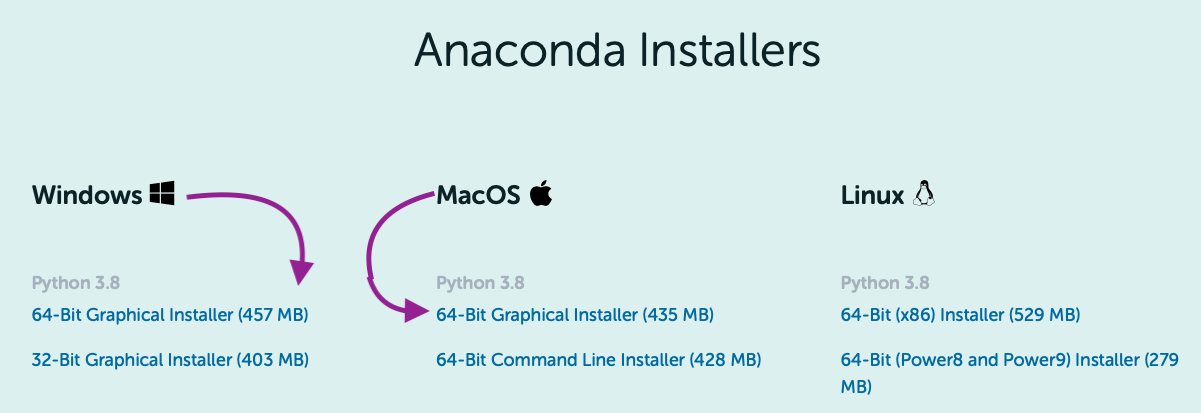NB: If you are one of those few still working with Python 2.7 (I was one until last fall), worry not, I will show you how to create a Python 2.7 virtual environment without much effort.

#### Step 2 – Install Anaconda

Follow the official installation instructions to the letter, with the exception of step 8. Here I would suggest especially if you want the ability to start JupyterLab from the command prompt, the alternative setting:NB: I realize this is discouraged because it may cause interference with other software down the road, but I’ve found no issue yet (not a guarantee, of course, so do at your own peril), and it is much easier than having to add the path manually.

#### Step 3 – Set Chrome as web browser for JupyterLab

I’ve never been able to make JupyterLab work with Internet Explorer, so this is not an optional step for me. To set Chrome as the browser for JupyterLab, open the config file `jupyter_notebook_config.py` (located in your home directory, in `~/.jupyter`), find the browser section:then replace the last line, `c.NotebookApp.browser = ''` , with:

`c.NotebookApp.browser = '"C:/path/to/your/chrome.exe" %s'` for example:

`‘”C:\Program Files\Google\Chrome\Application\chrome.exe” %s’`

N.B. For mac users, use this:

``c.NotebookApp.browser = 'open -a /Applications/Google\ Chrome.app %s'``

If the config file is not present in the home directory, it can be created at the prompt with the command:

``jupyter notebook --generate-config``

In Windows, this will create the file at this location:

`C:\Users\yourusername\.jupyter\jupyter_notebook_config.py`

#### Step 4 – Install nb_condas_kernels

The package nb_conda_kernels will later detect all conda environments that have notebook kernels and automatically registers them. As a result, all those environments will be visible and can be used directly from the JupyterLab interface. So:

a) check if `nb_conda_kernels` is installed by executing `conda list` at the prompt.

b) If you do not see it in the list of packages, then execute `conda install -c anaconda nb_conda_kernels`

#### Step 5 – Edit the Conda configuration file to create environments with default packages

To automatically install specific packages every time a new environment is created, add the package list to the create_default_packages section of the`.condarc` configuration file, which is located in the home directory. For example, in Windows, this would be:

`C:\Users\yourusername\.condarc`

If the `.condarc` configuration file is not present, you can:

• generate one using`conda config`, for example with:
``conda config --add channels conda-forge``

then edit it. The syntax for the file is the same used in environment files; here’s an example:NB:  `ipykernel` is necessary if `nb_conda_kernels` is to detect the environments.

If you’d prefer to install each package individually, you can install `ipykernel` with:

`conda install ipykernel`

#### Step 6 – Create the desired environments

To create both Python 3.6 and a Python 2.7 environments, for example, execute at the prompt the two commands below:

` conda create -n py37 python=3.7`

`conda create -n py27 python=2.7`

#### Step 7 – Start JupyterLab

At the prompt, execute the command:

`Jupyter lab`

This will open the JupyterLab Interface automatically in Chrome. From there you can select File>New>Notebook and you will be prompted to select a Kernel as below, where you see that both environments just created are available:#### Step 8 – Have fun

You are all set to work with the Notebooks in JupyterLab.

PS: To ensure the packages defined in the Conda configuration file were included in both environments, I run this example from the Seaborn tutorial: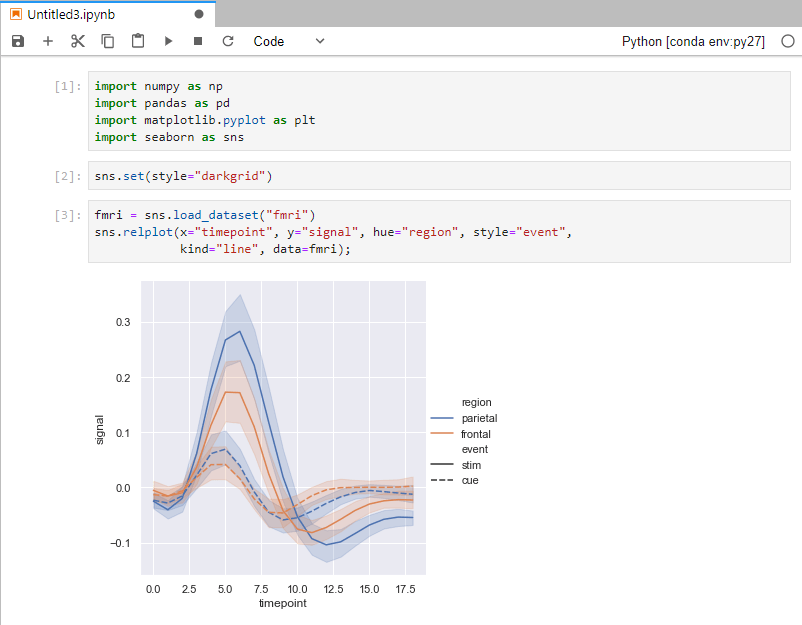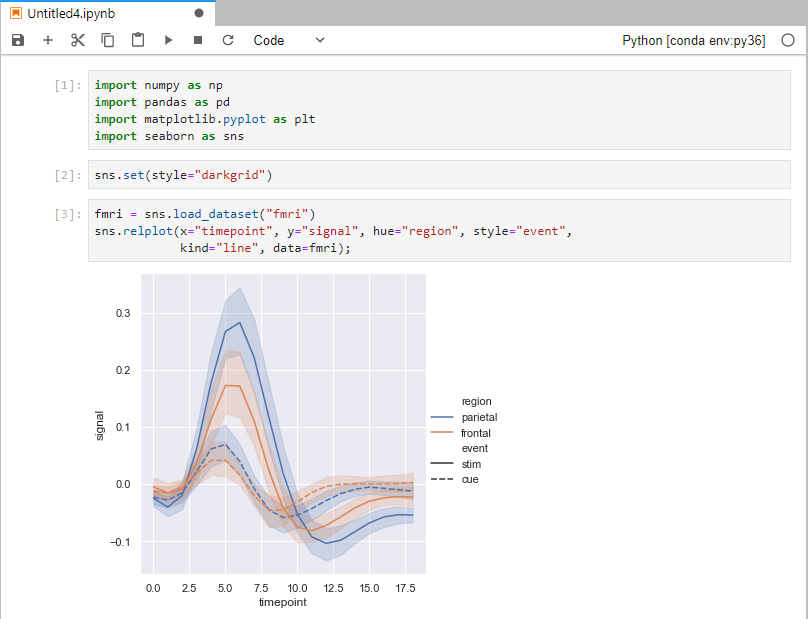# Geoscience Machine Learning bits and bobs – data inspection

If you have not read Geoscience Machine Learning bits and bobs – introduction, please do so first as I go through the objective and outline of this series, as well as a review of the dataset I will be using, which is from the  2016 SEG Machine LEarning contest.

#### *** September 2020 UPDATE ***

Although I have more limited time now, compared to 2016,  I am very excited to be participating in the 2020 FORCE Machine Predicted Lithology challenge. Most new work and blog posts will be about this new contest instead of the 2016 one.

#### ***************************

OK, let’s begin!

With each post, I will add a new notebook to the GitHub repo here. The notebook that goes with this post is  called 01 – Data inspection.

#### Data inspection

The first step after loading the dataset is to create a Pandas DataFrame. With the `describe` method I get a lot of information for free:Indeed, from the the first row in the summary I learn that about 20% of samples in the photoelectric effect column PE are missing.

I can use `pandas.isnull` to tell me, for each well, if a column has any null values, and `sum` to get the number of null values missing, again for each column.

```for well in training_data['Well Name'].unique():
print(well)
w = training_data.loc[training_data['Well Name'] == well]
print (w.isnull().values.any())
print (w.isnull().sum(), '\n')
```

Simple and quick, the output tells met, for example, that the well ALEXANDER D is missing 466 PE samples, and Recruit F9 is missing 12.

However,  the printout is neither easy, nor pleasant to read, as it is a long list like this:

```SHRIMPLIN
False
Facies       0
Formation    0
Well Name    0
Depth        0
GR           0
ILD_log10    0
DeltaPHI     0
PHIND        0
PE           0
NM_M         0
RELPOS       0
dtype: int64

ALEXANDER D
True
Facies         0
Formation      0
Well Name      0
Depth          0
GR             0
ILD_log10      0
DeltaPHI       0
PHIND          0
PE           466
NM_M           0
RELPOS         0
dtype: int64

Recruit F9
True
Facies        0
Formation     0
Well Name     0
Depth         0
GR            0
ILD_log10     0
DeltaPHI      0
PHIND         0
PE           12
NM_M          0
RELPOS        0
dtype: int64
...
...
```

From those I can see that, apart from the issues with the PE log, GR has some high values in SHRIMPLIN, and so on…

All of the above is critical to determine the data imputation strategy, which is the topic of one of the next posts; but first in the next post I will use a number of visualizations of  the data, to examine its distribution by well and by facies, and to explore relationships among variables.

# Geoscience Machine Learning bits and bobs – introduction

#### Bits and what?

After wetting (hopefully) your appetite with the Machine Learning quiz / teaser I am now moving on to a series of posts that I decided to title “Geoscience Machine Learning bits and bobs”.

OK, BUT fist of all, what does ‘bits and bobs‘ mean? It is a (mostly) British English expression that means “a lot of small things”.

Is it a commonly used expression? If you are curious enough you can read this post about it on the Not one-off British-isms blog. Or you can just look at the two Google Ngram plots below: the first is my updated version of the one in the post, comparing the usage of the expression in British vs. US English; the second is a comparison of its British English to that of the more familiar “bits and pieces” (not exactly the same according to the author of the blog, but the Cambridge Dictionary seems to contradict the claim).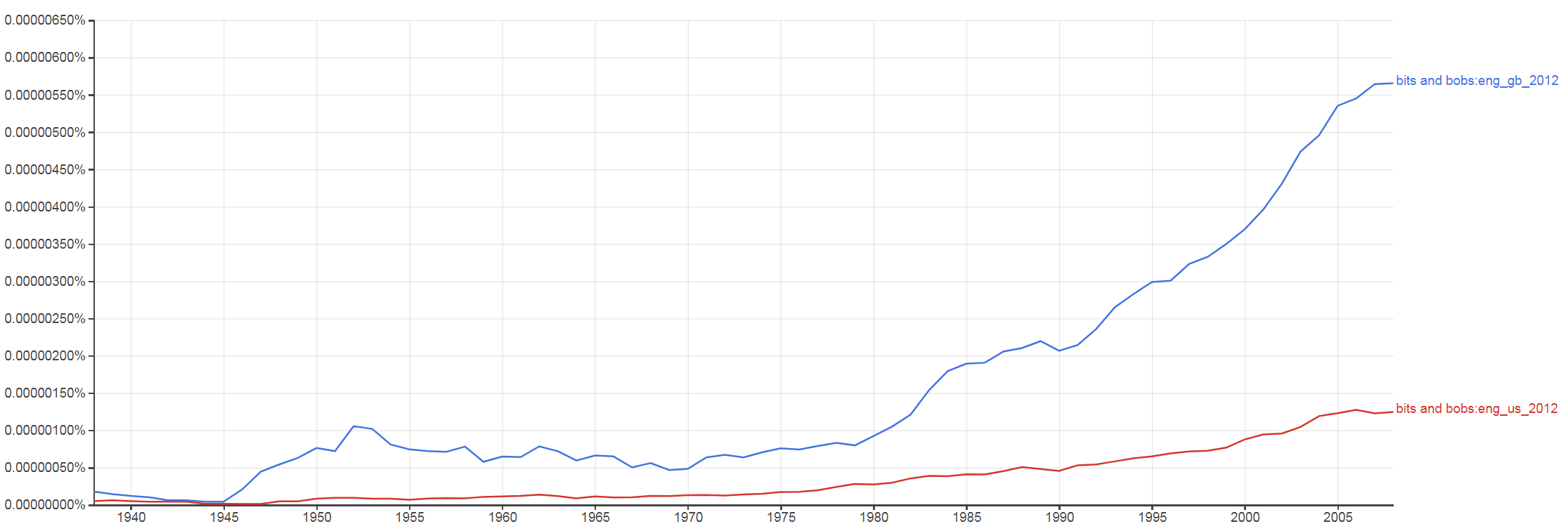I’ve chosen this title because I wanted to distill, in one spot, some of the best collective bits of Machine Learning that came out during, and in the wake of the 2016 SEG Machine Learning contest, including:

• The best methods and insights from the submissions, particularly the top 4 teams
• Things that I learned myself, during and after the contest
• Things that I learned from blog posts and papers published after the contest

I will touch on a lot of topics but I hope that – in spite of the title’s pointing to a random assortment of things –  what I will have created in the end is a cohesive blog narrative and a complete, mature Machine Learning pipeline in a Python notebook.

#### *** September 2020 UPDATE ***

Although I have more limited time these days, compared to 2016,  I am very excited to be participating in the 2020 FORCE Machine Predicted Lithology challenge. Most new work and blog posts will be about this new contest instead of the 2016 one.

#### Some background on the 2016 ML contest

The goal of the SEG contest was for teams to train a machine learning algorithm to predict rock facies from well log data. Below is the (slightly modified) description of the data form the original notebook by Brendon Hall:

The data is originally from a class exercise from The University of Kansas on Neural Networks and Fuzzy Systems. This exercise is based on a consortium project to use machine learning techniques to create a reservoir model of the largest gas fields in North America, the Hugoton and Panoma Fields. For more info on the origin of the data, see Bohling and Dubois (2003) and Dubois et al. (2007).

This dataset is from nine wells (with 4149 examples), consisting of a set of seven predictor variables and a rock facies (class) for each example vector and validation (test) data (830 examples from two wells) having the same seven predictor variables in the feature vector. Facies are based on examination of cores from nine wells taken vertically at half-foot intervals. Predictor variables include five from wireline log measurements and two geologic constraining variables that are derived from geologic knowledge. These are essentially continuous variables sampled at a half-foot sample rate.

The seven predictor variables are:

The nine discrete facies (classes of rocks) are:#### Tentative topics for this series

• List of previous works (in this post)
• Data inspection
• Data visualization
• Data sufficiency
• Data imputation
• Feature augmentation
• Model training and evaluation
• Connecting the bits: a full pipeline

#### List of previous works (comprehensive, to the best of my knowledge)

In each post I will make a point to explicitly reference whether a particular bit (or a bob) comes from a submitted notebook by a team, a previously unpublished notebook of mine, a blog post, or a paper.

However, I’ve also compiled below a list of all the published works, for those that may be interested.

The Github repo with all teams’ submissions.

Two blog posts by Matt Hall of Agile Scientific, here and here

The published summary of the contest by Brendon Hall and Matt Hall on The Leading Edge

An SEG extended abstract on using gradient boosting on the contest dataset

An arXiv e-print paper on using a ConvNet on the contest dataset

Abstract for a talk at the 2019 CSEG / CSPG Calgary Geoconvention

# Variable selection in Python, part I

#### Introduction and recap

In my previous two posts of this (now official, but) informal Data Science series I worked through some strategies for doing visual data exploration in Python, assisted by domain knowledge and inferential tests (rank correlation, confidence, spuriousness), and then extended the discussion to more robust approaches involving distance correlation and variable clustering.

For those that have not read those posts, I am using a dataset comprising 21 wells producing oil from a marine barrier sand reservoir; the data was first published by Lee Hunt in 2013 in a CSEG Recorder paper titled Many correlation coefficients, null hypotheses, and high value.

Oil production, the dependent variable, is measured in tens of barrels of oil per day (it’s a rate, actually). The independent variables are: Gross Pay, in meters; Phi-h, porosity multiplied by thickness, with a 3% porosity cut-off; Position within the reservoir (a ranked variable, with 1.0 representing the uppermost geological facies, 2.0 the middle one, 3.0 the lowest one); Pressure draw-down in MPa. Three additional ‘special’ variables are: Random 1 and Random 2, which are range bound and random, and were included in the paper, and Gross Pay Transform, which I created specifically for this exercise to be highly correlated to Gross pay, by passing Gross pay to a logarithmic function, and then adding a bit of normally distributed random noise.

#### Next step: variable selection (Jupyter Notebooks here)

The idea of variable selection is to try to understand which independent variables are more and which are less important in predicting the dependent variable (Production in this case), and also which ones may be highly correlated to one another (in other words, they carrying the same information); in both cases, assisted by domain knowledge, we drop some of the variables, resulting (ideally) in an improved prediction by a model that is simpler and can generalize better.

I really love the systematic way in which Thomas, working on the same dataset but using R, looked at several methods for variable selection and then summarized all the results in a table. The insight from this (quite) exhaustive analysis helped him chose a subset of variables to use in the final regression. I really, REALLY recommend reading his interactive R notebook.

As for me, one of the goals I had in mind at the end of our 2018 collaboration on this project was to be able to do something similar in Python, and I am delighted to say I think I was able to achieve that goal.

In this post I will look at:

• Distance correlation, again
• Multicollinearity, using Variance Inflation Factor (VIF)
• Sequential feature selection, using both a backward and forward approach
• Random Forest Regressor variable importance, using a drop-column approach
• Multicollinearity, using variable dependence

In the next (1 or 2) post(s) I will look at:

• Permutation importance using an Extra Tree Regressor
• Mutual information
• The relative magnitude of the transformed variables in ACE (Alternating Conditional Expectation)
• SHAP values (Shapley additive explanations)
• The sign of the weights of a neural network (excitory (positive weights) vs. inhibitory (negative weighs))

I think this is a good mix as it combines methods and then summarize the results from all methods.

##### Distance correlation

in Figure 1, below, I plot again the correlation matrix of bivariate scatterplots, rearranged according to the clustering results from last post, and with the distance correlation annotated and coloured by its bootstrapping p-value.

Phi-h, Gross Pay, and Gross pay transform are highly correlation to Production, with statistical significance at the 10%level given by the p-value. However, there is a good chance also also of multicollinearity at play, almost certainly between Gross Pay and Gross Pay Transform, with a DC of 0.97; we know why, in this case, imposed it in this case, but we might have not known.Figure 1. Seaborn pairgrid matrix with distance correlation colored by p-value (gray if > 0.10, blue if p <= 0.10), and plots rearranged by clustering results

##### Variance Inflation Factor (VIF)

Variance inflation factor (VIF) is a technique to estimate the severity of multicollinearity among independent variables within the context of a regression. It is calculated as the ratio of all the variances in a model with multiple terms, divided by the variance of a model with one term alone.

First, we set-up a regression, using the Patsy library to generate design matrices for target and predictors:

```outcome, predictors = dmatrices("Production ~ Gross_pay +Phi_h +Position
+Pressure +Random1 +Random2 +Gross_pay_transform",
data, return_type='dataframe')
```

for which then VIF factors can be calculated with:

```vif["VIF Factor"] = [variance_inflation_factor(predictors.values, i)
for i in range(predictors.shape)]```

The values are summarized in Table I below; variables that have variance inflation factor that is high (ignoring the intercept) and similar in value have a high chance of being collinear because they explain the same variance in the dataset.Table I. Regression VIF factors

For this model, the result suggests either Gross Pay or Gross Pay Transform should be dropped, otherwise the risk is of building a model with high multicollinearity (that is, predictions would be very susceptible to small noise fluctuations).

But which one should we drop? It occurred to me that one possibility would be to drop one in turn and recalculate the VIF factors.Table II. VIF after dropping Gross Pay Transform

As seen in Table II, after removing Gross Pay Transform  all VIF factors are below the cut-off value of 5 (rule-of-thumb suggested in this article, and reference therein). I  would make the additional observation. that because the factors for Phi-h and Gross Pay are now close, even though below the cutoff,  there may be some (smaller amount of) collinearity between the two variables, which is consistent to be expected since both variables contain some information on height (one of pay, one of porosity).

We see something similar when removing Gross Pay; in fact, the Factors for Gross Pay Transform and Phi-h in Table III are also close, yes, but smaller. I’d conclude that VIF is veru sueful in highlighting multicollinearity, but it does not necessarily answer the question of which collinear feature shoud be dropped.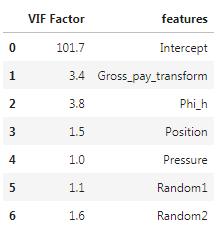Table III. VIF after dropping Gross Pay

##### Sequential feature selection

Sequential feature selection (similarly to Scikit-learn’s Recursive Feature Elimination) is used  “to automatically select a subset of features that is most relevant to the problem. The goal of feature selection is two-fold: we want to improve the computational efficiency and reduce the generalization error of the model by removing irrelevant features or noise”.

I tested both Sequential Forward Selection (SFS) and Sequential Backward Selection (SBS) from Sebastan Raschka‘s  `mlxtend` library to search for that optimal subset of features (for a full overview of the method, and a great set of detailed examples, please see the excellent documentation by Sebastian). You can download and run the full notebook fro the GitHub repo here).

The only difference between SFFS and SBFS is that the former starts with at 1 feature and adds them one by one, whereas the latter starts with all features (or a user defined pre-selected number) and removes them one by one. In both cases I used the selector as part of a pipeline including Scikit-learn’s linear regression and cross-validation with Leave One Out (i.e., dropping one well at a time); for example, the pipeline for SFS is:

```features = data.loc[:, ['Position', 'Gross pay', 'Phi-h', 'Pressure',
'Random 1', 'Random 2', 'Gross pay transform']].values
y = data.loc[:, ['Production']].values

LR = LinearRegression()
loo = LeaveOneOut()

sfs = SFS(estimator=LR,
k_features=7,
forward=True,
floating=True,
scoring='neg_mean_squared_error',
cv = loo,
n_jobs = -1)
sfs = sfs.fit(X, y)
```

and the feature selection results are plotted in Figure 2, generated with a modified version of Sebastian’s `mlxtend` utility function:

```plot_sequential_feature_selection(sfs.get_metric_dict(), kind='std_err')
plt.gca().invert_yaxis()
```

Please notice that having flipped the y axis (my personal preference), performance for SFFS (as given by negative mean square error) improves towards the bottom.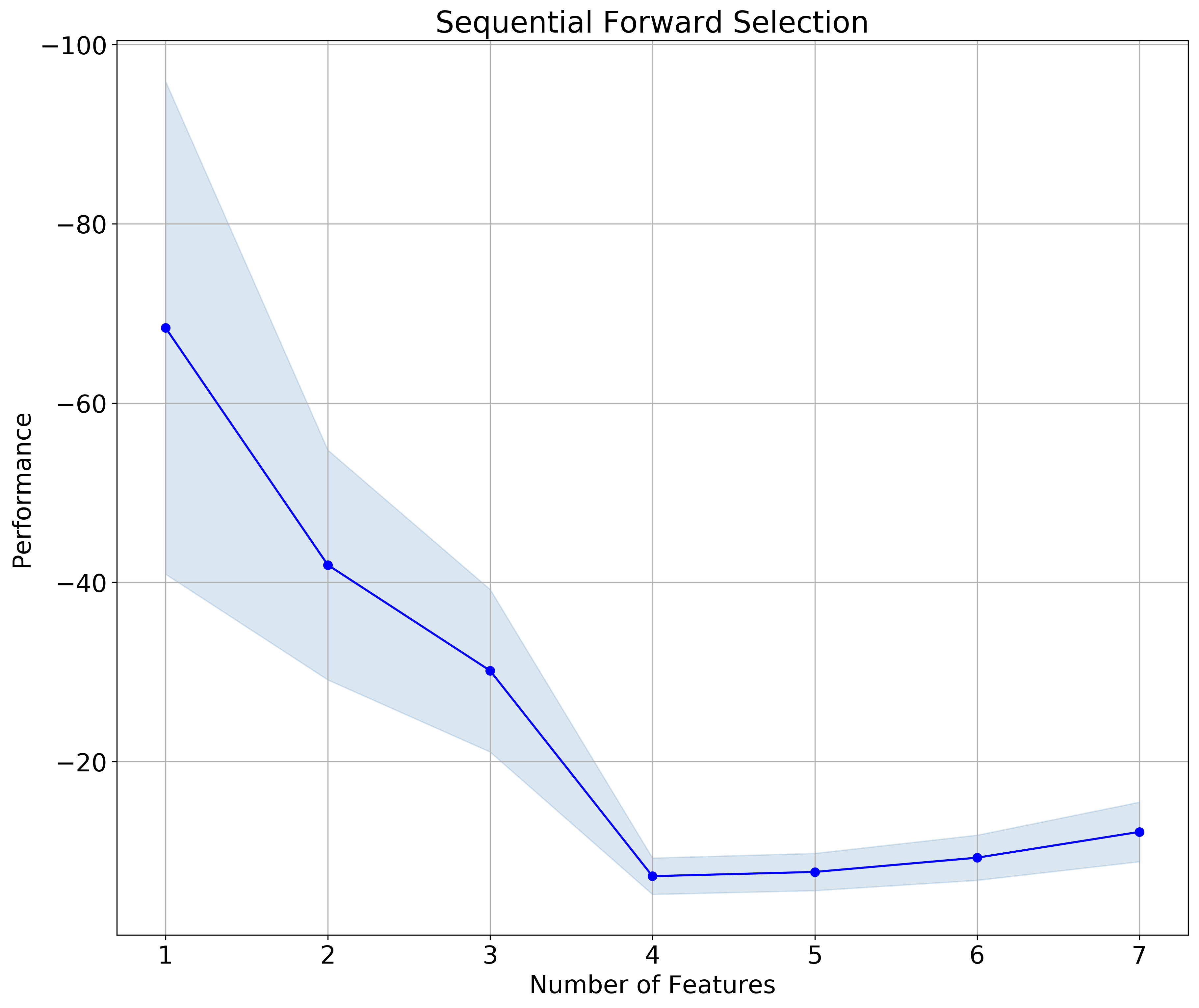Figure 2. Sequential Forward Selection

The results for SFBS is plotted in Figure 3. Notice that in this case I flipped both the y axis  and the x axis; the latter makes the sequential selection go from left to right, which I find a bit more intuitive, given we read from left to right.Figure 3. Sequential Backward Selection

In both cases the subset is made up of 4 feature, and – to my delight !! –  the selected features are the same (check the notebook to see how I extract the information):

```>>>  ['Position', 'Gross pay', 'Phi-h', 'Pressure']
```
##### Drop-column feature importance

You can download the notebook for both drop-column importance and dependence from here.

I have to say I’ve never been comfortable with using Feature Importance plots you get from Random Forest. In part because, on occasion, I noticed a disconnect with what domain knowledge-informed intuition would suggest; in part, I confess, because I thought (and I was right) I had an incomplete understanding of what goes on in the background. Until I read the article How to not use random forest. The example with toy dataset in there is not the most exciting, but it demonstrate clearly how using Feature Importance with preset parameters places a random variable at the top. If you wonder how can that be, I recommend reading the article.

Or read on, there’s more coming: curious, I did some more searching, and found this article, Selecting good features – Part III: random forests. There’s a nicer example in there, using the Boston Housing dataset, and to me a clearer explanation of why one should not use the default Scikit-learn Mean Decrease Impurity metric (strong, but correlated features can end up with low scores).

Finally, I found Beware Default Random Forest Importances, where the authors (thank you!!!) not only walk readers through a full set of experiments, run in both Python and R, but provide a great library (called `rfpimp`), to do your own work in Python.

I really like their drop-column importance, which is implemented to answers the question of how important a feature is to the overall model performance … and does it  … even more directly than the permutation importance.

That is achieved with a brute force drop-column apprach involving:

• training the model with all features to get a baseline performance score
• dropping a column
• retraining  the model and recomputing the performance score.

The importance value of a feature is then the difference between the baseline and the score from the model without that feature.

I also REALLY like that unimportant features do not have just very low importance; some do, but some have negative importance, exposing that removing them improves model performance. This is the case, with our small dataset of the Random 1 and Random 2 variables, as shown in Figure 4. It is also the case of Pressure. Of the remaining variables, Gross Pay Transform has very low importance (please notice the range is 0-0.15 for this plot, a conscious choice by the authors), Gross pay and Phi-h look somewhat important, and Position in the reservoir is the most important feature. This is excellent insight; please compare to the importances with Scikit-learn’s defautl metric, in Figure 5.Figure 4. rfpimp Drop-column importance. Notice the 0-0.15 range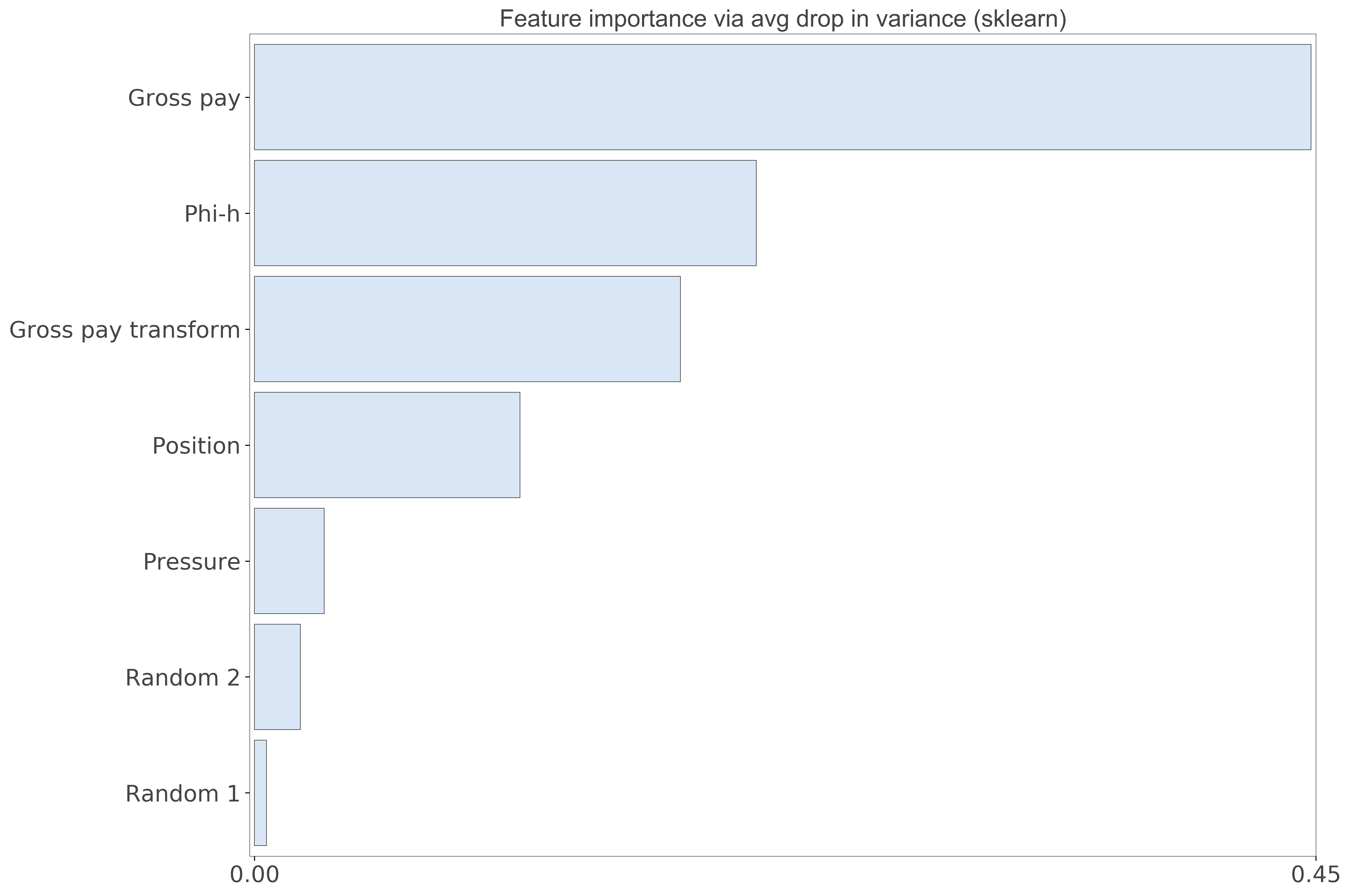Figure 5. Scikit-learn Feature importance. Notice the 0-0.45 range

##### Dependence

This last analysis is similar to Thomas’ Redundancy Analysis in that we look for those variables that can be predicted using the other variables.  Using the `feature_dependence_matrix` function from the `rfpimp` library we get:

```>>> Dependence:
Gross pay               0.939
Gross pay transform     0.815
Phi-h                   0.503
Random 2               0.0789
Position               0.0745
Pressure               -0.396
Random 1               -0.836

```

By removing Gross Pay Transform, and repeating the analysis, we get:

```>>> Dependence:
Gross pay    0.594
Phi-h        0.573
Random 2     0.179
Position     0.106
Pressure    -0.339
Random 1    -0.767```

and by removing Gross Pay:

```>>> Dependence:
Gross pay transform     0.479
Phi-h                   0.429
Position                0.146
Random 2              -0.0522
Pressure               -0.319
Random 1               -0.457

```

These results show, again, that either Gross Pay or Gross Pay Transform should be dropped (perhaps the former), because of very high chance of dependence (~multicollinearity). Also Phi-h is somewhat predictable from the other variables, but not as much, so it may be fine, if not good, to keep it (that’s what domain knowledge would suggest).

They are in agreement with the results from VIF, but this time the outcome is blind to the outcome (the target Production) so I’d consider it more robust.

# Having fun learning Python with your kids

When I was a kid I loved video games. Now … we’re talking about 70s and 80s games: my first home computer was a Texas Instruments TI-99/4A, my second one a Commodore 64. I loved all games in those days, no matter how primitive they might seem like now; you can get a glimpse of WHAT we are talking about in this video. And funny enough, I still really do love them; below is one of my favorites, H.E.R.O. which I can play these days thanks to a C64 emulator.Unfortunately for me, I did not catch (not then, at least) the programming bug. What really turned me down was having tried to type thousands of lines of BASIC to run what looked like an awesome adventure game from a command list in a magazine, only to never be able to play the game because the list was full of typos.

So, I want to make it better for both my daughter, AND myself,  because I plan to make more games. First of all, we are working with Python. There are a lot of resources for making games in Python; a really good one is Al Sweigart‘s Invent Your Own Computer Games with Python (as an aside: I have been using a chapter here, a chapter there from many of his books, it is awesome that he made them available online, but it was time to give back so I bought the 4th edition of Invent Your Own Computer Games with Python).

To set the stage for our first game I reviewed the first few chapters of the book, particularly Chapter 5: Dragon Realm, to get some ideas on how to use Python to make interactive games, but then decided to start with our own game: a calculator that would talk to the player, via the terminal, and ask for numbers and for their favorite algebraic operation. However, we added a twist because it sounded a lot more fun: IF her mom were to play, we’d want the results to be occasionally wrong. My daughter worked out some of the logic, after suggesting the twist, and I did the heavy lifting with Python; in the remainder of the post I will work through how we did it. Please note this game is written for Python 3.

In the first block, Jester, the calculator, introduces itself and then asks the player for their name; the name is stored to a variable via the `input` function and used for greeting and throughout the game. The `capitalize()` method turns the first letter of the name string to upper case, if needed.

```print ('Hello, I am a talking calculator and my name is Jester.')
name = input('What is your name? ')
print ('Hello ' + name.capitalize() + ', nice to meet you!')```

The real game starts in the big block below:

```while True:
operation = input(name.capitalize() +
', would you like to add or subtract? (enter "a" or "s"; "q" to quit) ')
if operation.lower() =='q':
print('Goodbye ' + name.capitalize())
break
elif operation.lower() =='s' or operation.lower() =='a':
input1 = input('Can I have the first number? ')
try:
num1=int(input1)
except ValueError:
print ("That is not a number!")
input1 = input('Can I have the first number? ')
input2 = input('Can I have the second number? ')
try:
num2=int(input2)
except ValueError:
print("That is not a number!")
input2 = input('Can I have the second number? ')
num1=int(input1)
num2=int(input2)```

Let’s break it up. First, the player chooses an operation (addition or subtraction) again via the `input` function. The `while` loop allows the player to continue playing after each operation.

```while True:
operation = input(name.capitalize() +
', would you like to add or subtract? (enter "a" or "s"; "q" to quit) ')```

The following `if` statement is used to exit the `while` loop (using `break`) if the player decides to quit.

```  if operation.lower() =='q':
print('Goodbye ' + name.capitalize())
break```

An `elif` statement comes next, allowing the player to chose the operation; at the same time if neither ‘a’ nor ‘s’ are chosen, the program will go back to the beginning of the `while` loop.

```  elif operation.lower() =='s' or operation.lower() =='a':

Finally, Jester asks for the first number to be added or subtracted. We want to be sure that the string passed by the user in here is a number, so we use a `try` clause to handle a possible `exception` (for example a letter is entered instead of a number). Here is the logic: if when prompted for the first number we typed a letter by accident (for example the string ‘w’, instead of the string ‘3’ , when `input1`  is passed to `int` we’d get this fatal error and the program would be interrupted:

```>>> num1=int(input1)
>>> ValueError: invalid literal for int() with base 10: 'w'```

With the `try` clause we instead handle the `exception` by printing the custom error message “That is not a number!“, followed by a new request for a number:

```    input1 = input('Can I have the first number? ')
try:
num1=int(input1)
except ValueError:
print ("That is not a number!")
input1 = input('Can I have the first number? ')
num1=int(input1)```

The above cycle is repeated for the second number. After Jester gets two legitimate integers to work with, the operation part begins, which includes Jester’s prank. Indeed, depending on the name of the player, the result will be either always correct, or sometimes correct, sometimes a bit off (which is done using `random`). We picked “Olivia” to represent the “lucky” object of the prank. This is the only hard-coded part of the program. It needs to be changed with the name of the intended “victim”.

There are two nested `if` statements below that allow for the following to happen: `if` the player’s name is Olivia, a random number between 1 and 3, `rand1` is drawn.  Then the  value of `rand1` is checked: if `if` it is 3 then a non-zero number, `num3`, of value drawn between 1 and 5 (`rand2`) will be added to the result of the operation to make it wrong; otherwise the operation will execute correctly.

```from random import randint
num3 = 0 # initializes random error variable
if (name.lower() == 'olivia'): # for this player ....
rnd1 = randint(1,3)
rnd2 = randint(1,5)
if rnd1 == 3: # .... if a 3 is drawn the correct result
num3 = rnd2 # .... will be modified by this random amount```

Finally, this is the addition and subtraction block: in both cases unless “Olivia” is the player `num3` will be zero and the result will be correct.

```  if operation.lower() == 'a':
result = num1 + num2 + num3
print (str(num1) + ' + '  + str(num2) + ' = ' + str(result))
elif operation.lower() == 's':
result = (max(num1, num2) - min(num1, num2)) - num3
print (str(max(num1, num2)) + ' - '
+ str(min(num1, num2))  + ' = ' + str(result))```

If you want to play the game, please copy the full program below and paste it in a .py file and give it a try. Enjoy!

```# NB optimized for Python 3
from random import randint

print ('Hello, I am a talking calculator and my name is Jester.')
name = input('What is your name? ')
print ('Hello ' + name.capitalize() + ', nice to meet you!')

while True:
operation = input(name.capitalize() +
', would you like to add or subtract? (enter "a" or "s"; "q" to quit) ')

if operation.lower() =='q':
print('Goodbye ' + name.capitalize())
break

elif operation.lower() =='s' or operation.lower() =='a':
input1 = input('Can I have the first number? ')
try:
num1=int(input1)
except ValueError:
print ("That is not a number!")
input1 = input('Can I have the first number? ')

input2 = input('Can I have the second number? ')
try:
num2=int(input2)
except ValueError:
print("That is not a number!")
input2 = input('Can I have the second number? ')

num1=int(input1)
num2=int(input2)

num3 = 0
if (name.lower() == 'olivia'):
rnd1 = randint(1,3)
rnd2 = randint(1,5)
if rnd1 == 3:
num3 = rnd2

if operation.lower() == 'a':
result = num1 + num2 + num3
print (str(num1) + ' + '  + str(num2) + ' = ' + str(result))

elif operation.lower() == 's':
result = (max(num1, num2) - min(num1, num2)) - num3
print (str(max(num1, num2)) + ' - '
+ str(min(num1, num2))  + ' = ' + str(result))```

# Machine Learning in Python: classification using Support Vector Machines and Scikit-learn

This post is a short extract, with minor modifications,  from my recently released article on the check the CSEG Recorder Machine Learning in Geoscience V: Introduction to Classification with SVMs.

### Understanding classification with Support Vector Machines

Support Vector Machines are a popular type of algorithm used in classification, which is the process of  “…identifying to which of a set of categories (sub-populations) a new observation belongs (source: Wikipedia).

In classification, the output variable is a category, for example ‘sand’, or ‘shale’, and the main task of the process is the creation of a dividing boundary between the classes. This boundary will be a line in a bi-dimensional space (only two features used to classify), a surface in a three dimensional space (three features), and a hyper-plane in a higher- dimensional space. In this article I will use interchangeably the terms hyper-plane, boundary, and decision surface.

Defining the boundary may sound like a simple task, especially with two features (a bidimensional scatterplot), but it underlines the important concept of generalization, as pointed out by Jake VanderPlas in his Introduction to Scikit-Learn, because ”… in drawing this separating line, we have learned a model which can generalize to new data: if you were to drop a new point onto the plane which is unlabeled, this algorithm could now predict…” the class it belongs to.

Let’s use a toy classification problem to understand in more detail how in practice SVMs achieve the class separation and find the hyperplane. In the figure below I show an idealized version (with far fewer points) of a Vp/Vs ratio versus P-impedance crossplot from Amato del Monte (2017, Seismic rock physics, tutorial on The Leading Edge).  I’ve added three possible boundaries (dashed lines) separating the two classes.

Each boundary is valid, but are they equally good? Well, for the SVM classifier, they are not because the classifier looks for the boundary with the largest distance from the nearest point in either of the classes.

These points, called Support Vectors, are the most representative of each class, and typically the most difficult to classify. They are also the only ones that matter; if a Support Vector is moved, the boundary will also move. However, if any other point is moved, provided that it is not moved into the margin or across the boundary, it would have no effect on the boundary. This makes SVM classifiers insensitive to outliers (points very far away from the rest of the points in their class and from the boundary) and also less memory intensive than other classifiers (for example, the perceptron). The process of finding this boundary is referred to as “maximizing the margin”, where the margin is a corridor with no data points between the boundary and the support vectors. The larger this buffer, the lower the generalization error; conversely, small margins are almost invariably associated with over-fitting. We will see more on this in a subsequent section.

So, to go back to the question, which of the three proposed boundaries is the best one (and by “best” I am referring to the one that will generalize better to unseen data)? Based on what we’ve learned so far, it would have to be the green boundary. Indeed, the orange one is so close to its support vectors (the two points circled with orange) that it leaves virtually no margin; the purple boundary is slightly better (the support vectors are the points circled with purple) but its margin is still quite small compared to the green boundary.

Maximizing the margin is the goal of the SVM classifier, and it is a constrained optimization problem. I refer interested readers to Hearst (1998, Support Vector Machines, IEEE Intelligent Systems); however, I will quote a definition from that paper (with reference to Figure 1 and accompanying text) as it yields further understanding: “… the optimal hyper-plane is orthogonal to the shortest line connecting the convex hulls of the two classes, and intersects it half way”.

In the inset in the figure, I zoomed closer to the 4 points near the green boundary; I’ve also drawn the convex hulls for the classes, the margin, and the shortest orthogonal line, which is bisected by the hyper-plane. I have selected (by hand) the best hyper-plane already (the green one), but if you can imagine rotating a line to span all possible orientations in the empty space close to the two classes without intersecting either of the hulls and find the one with the largest margin, you’ve just done quadratic optimization in your head. Moreover, you’ve turned a crossplot into a decision surface (quoted from Sebastian Thrun,  Intro to Machine Learning, Udacity 120 course).

If you are interested in learning more about Support Vector Machines in an intuitive way, and then how to try classification in practice (using Python and the Scikit-learn library), read the full article here, check the GitHub repo, then read How good is what? (blog post by Evan Bianco of Agile Scientific) for an example and DIY evaluation of  classifier performance.

# How to fix rainbows and other bad colormaps using Python

Yep, colormaps again!

In my 2014 tutorial on The Leading Edge I showed how to Evaluate and compare colormaps (Jupyter notebook here). The article followed an extended series of posts (The rainbow is dead…long live the rainbow!) and then some more articles on rainbow-like colormap artifacts (for example here and here).

Last year, in a post titled Unweaving the rainbow, Matt Hall described our joint attempt to make a Python tool for recovering digital data from scientific images (and seismic sections in particular), without any prior knowledge of the colormap. Please check our GitHub repository for the code and slides, and watch Matt’s talk (very insightful and very entertaining) from the 2017 Calgary Geoconvention below:

One way to use the app is to get an image with unknown, possibly awful colormap, get the data, and re-plot it with a good one.

Matt followed up on colormaps with a more recent post titled No more rainbows! where he relentlessly demonstrates the superiority of perceptual colormaps for subsurface data. Check his wonderful Jupyter notebook.

So it might come as a surprise to some, but this post is a lifesaver for those that really do like rainbow-like colormaps. I discuss a Python method to equalize colormaps so as to render them perceptual.  The method is based in part on ideas from Peter Kovesi’s must-read paper – Good Colour Maps: How to Design Them – and the Matlab function equalisecolormap, and in part on ideas from some old experiments of mine, described here, and a Matlab prototype code (more details in the notebook for this post).

Let’s get started. Below is a time structure map for a horizon in the Penobscot 3D survey (offshore Nova Scotia, licensed CC-BY-SA by dGB Earth Sciences and The Government of Nova Scotia). Can you clearly identify the discontinuities in the southern portion of the map? No?OK, let me help you. Below I am showing the map resulting from running a Sobel filter on the horizon.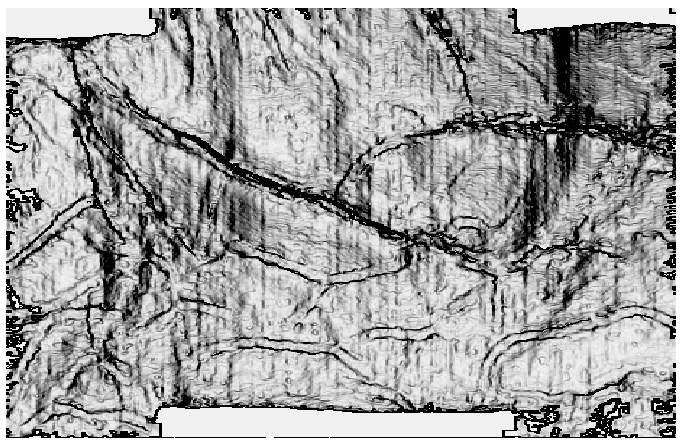This is much better, right? But the truth is that the discontinuities are right there in the original data; some, however, are very hard to see because of the colormap used (nipy spectral, one of the many Matplotlib cmaps),  which introduces perceptual artifacts, most notably in the green-to-cyan portion.

In the figure below, in the first panel (from the top) I show a plot of the colormap’s Lightness value (obtained converting a 256-sample nipy spectral colormap from RGB to Lab) for each sample; the line is coloured by the original RGB colour. This erratic Lightness profile highlights the issue with this colormap: the curve gradient changes magnitude several times, indicating a nonuniform perceptual distance between samples.

In the second panel, I show a plot of the cumulative sample-to-sample Lightness contrast differences, again coloured by the original RGB colours in the colormap. This is the best plot to look at because flat spots in the cumulative curve correspond to perceptual flat spots in the map, which is where the discontinuities become hard to see. Notice how the green-to-cyan portion of this curve is virtually horizontal!

That’s it, it is simply a matter of very low, artificially induced perceptual contrast.

Solutions to this problem: the obvious one is to Other NOT use this type of colormaps (you can learn much about which are good perceptually, and which are not, in here); a possible alternative is to fix them. This can be done by re-sampling the cumulative curve so as to give it constant slope (or constant perceptual contrast). The irregularly spaced dots at the bottom (in the same second panel) show the re-sampling locations, which are much farther apart in the perceptually flat areas and much closer in the more dipping areas.

The third panel shows the resulting constant (and regularly sampled) cumulative Lightness contrast differences, and the forth and last the final Lightness profile which is now composed of segments with equal Lightness gradient (in absolute value).Here is the structure map for the Penobscot horizon using the nipy spectum before and after equalization on top of each other, to facilitate comparison. I think this method works rather well, and it will allow continued use of their favourite rainbow and rainbow-like colormaps by hard core aficionados.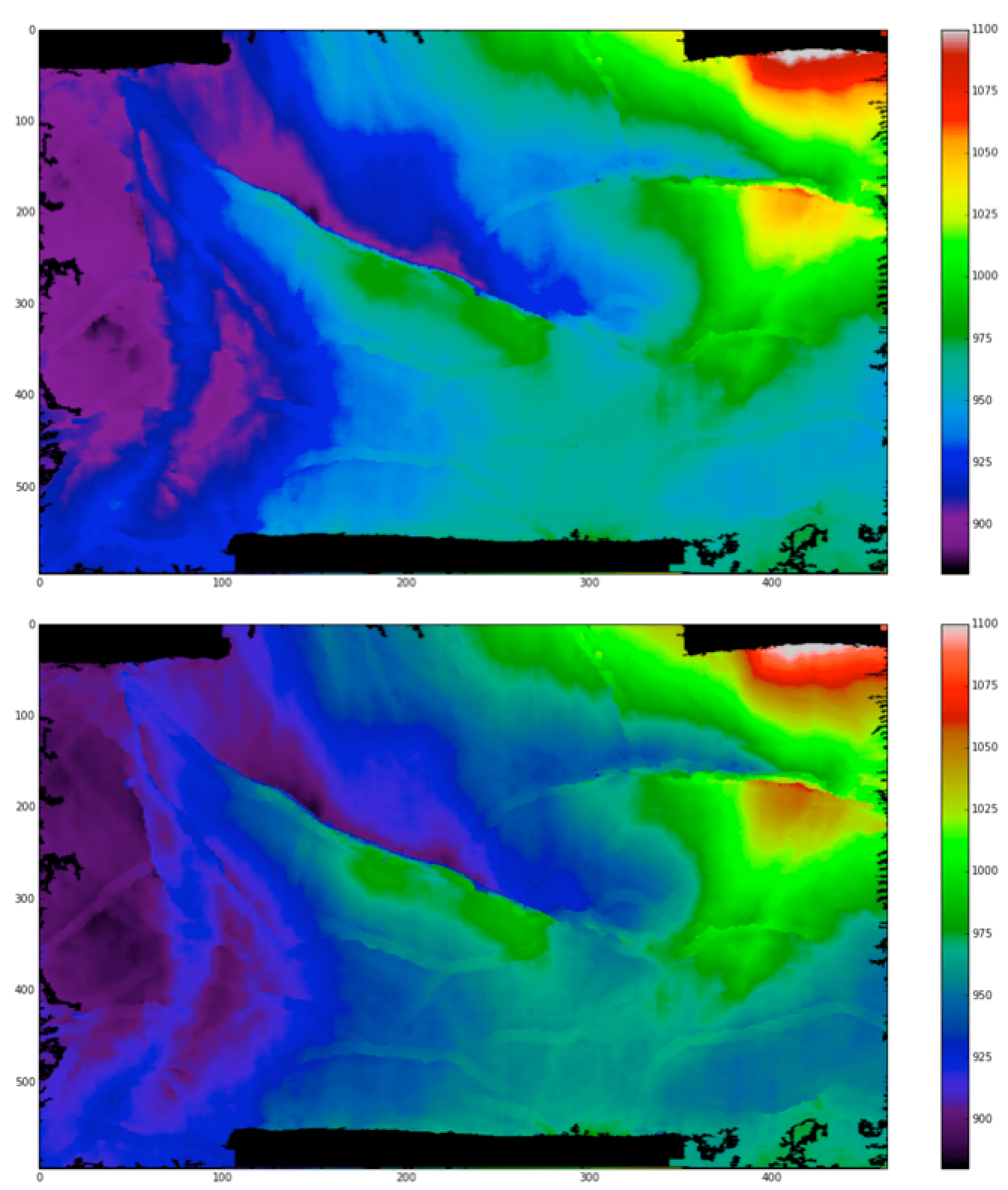If you want the code to try the equalization, get the noteboook on GitHub.